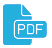# Author Details

## Sevastianov, Leonid A.

 Issue Section Title File Vol 27, No 3 (2019) Computational modeling and simulation On the properties of numerical solutions of dynamical systems obtained using the midpoint method(Eng) Vol 27, No 4 (2019) Mathematical Modeling Leaky waves in planar dielectric waveguide(Eng) Vol 27, No 4 (2019) Mathematical models in Physics Numerical modeling of stationary pseudospin waves on a graphene monoatomic films(Eng) Vol 28, No 1 (2020) Mathematical Modeling Numerical determination of the singularity order of a system of differential equations(Eng) Vol 28, No 4 (2020) Articles Solving the inverse problem for determining the optical characteristics of materials(Eng) Vol 30, No 1 (2022) Articles Finite-difference methods for solving 1D Poisson problem(Eng) Vol 30, No 2 (2022) Articles Complex eigenvalues in Kuryshkin-Wodkiewicz quantum mechanics(Eng) Vol 30, No 3 (2022) Articles Numerical simulation of cold emission in coaxial diode with magnetic isolation(Eng) Vol 31, No 2 (2023) Articles Chebyshev collocation method for solving second order ODEs using integration matrices(Eng)